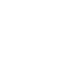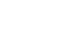2

# Data Structures and Algorithms Tutorial – Best Guide 2021

The first thing, when it comes to the field of Data structures and algorithms is to understand what it means, what are its uses and what are the various careers that you can opt for, with a Knowledge of Data structures and algorithms.

## Introduction to Data Structures and Algorithms.

Knowledge of data structures and algorithms is required of people who design and develop computer programs of any kind of systems software or applications software. Before learning about Data structures and algorithms lets us understand what is data.

## What is Data??

Data are simply values or set of values. A data item refers to a single unit of values.

### What are Data Structures??

A Data structure is an arrangement of data in a computer’s memory or disk. Data structures include Arrays, Stacks, Queues, linked lists, binary trees, and hash tables, and etc.

Data Structure = Organized Data + Allowed Operations.

## Data structures Operations

• Traversing: Accessing each record exactly once so that certain items in the record may be processed.
• Searching: Finding the location of the record with a given key value, or finding the locations of all records that satisfy one or more conditions.
• Inserting: Adding a new record to the structure.
• Deleting: Removing a record from the structure.

## Types of Data Structure

• Primitive Data Structures
• Non-primitive Data Structures

### Primitive Data Structures

Primitive data structure is a kind of data structure that stores the data of only one type.

Example: Integer, Float, Boolean, char.

#### char

• This datatype represents only one character, like N, n, 8 ,* ? etc.
• Char datatype takes only 1 byte of memory.
```char ch;   /* declare char type variable ch */
ch='A';    /* store A into ch */```

#### int

• This datatype stores an integer number.
• It takes 2 bytes of memory.
```int num;   /* declare num variable as int type */
num=5000;  /* store 5000 into num */```

#### float

• This datatype represents a number with decimal point.
• Float takes 2 bytes of memory.
```float sal;         /* declare sal as float type */
sal='78965.80';    /* store 78965.80 into sal */```

#### double

• This datatype represents a big float number.
• It takes 8 bytes of memory.
```double speed;   /* declare speed as double type variable */
speed=3.14e8;   /* store 3.1X10  power 8 into speed*/```

### Non-primitive data structure

Non-primitive data structure is a type of data structure which is a user-defined that stores the data of different types in a single entity.

Example: Linear and Non Linear.

#### Linear Data Structures

Data structure where data elements are arranged sequentially or linearly where the elements are attached to its previous and next adjacent in what is called a linear data structure.

Examples: Arrays, Stacks, Queues, and linked lists.

#### Non Linear Data Structures

Data structures where data elements are not arranged sequentially or linearly are called non-linear data structures.

Examples: Trees and Graph.

## Arrays

• A linear array is a list of a finite number n of homogeneous data elements of the same type such that the elements of the array are referenced respectively by an index set consisting of n Consecutive numbers and the elements of the array are stored respectively in successive memory locations.
• If we choose the name A for the Array, then the elements of A are denoted by subscript notation

a1, a2,a3,….. an or by the parenthesis notation A,A,A……A[n].

## Stack

• A stack is a list of elements in which an element may be inserted or deleted only at one end, called me top of the stack. This means, in particular, that elements are removed from a stack in the reverse order of that in which they were inserted into the stack. Special terminology is used for two basic operations associated with stacks:
1. (a) “Push” is the term used to insert an element into a stack.
2. (b) “Pop” is the term used to delete an element from a stack.

## Queue

• A queue is a linear list of elements in which deletions can take place only at one end, called the front, and insertions can take place only at the other end, called the rear. The terms “front” and “rear” are used in describing a linear list only when it is implemented as, a queue.
• Queues are also called first-in first-out (FIFO) lists, since the first element in a queue will be the first element out of the queue. In other words, the order in which elements enter a queue is the order in which they leave. This contrasts with stacks, which are lastin first-out (LIFO) lists.

• A linked list, or one-way list, is a linear collection of data elements, called nodes, where the linear order is given by means of pointers.
• That is, each node is divided into two parts:
1. The first part contains the information of the element, and
2. The second part, called the link field or next pointer field, contains the address of the next node in the list.

## Trees

• A general tree (sometimes called a tree) is defined to be a nonempty finite set T of elements, called nodes, such that:

(1) T contains a distinguished element R, called the root of T.
(2) The remaining elements of T form an ordered collection of zero or more disjoint trees T1,T2, . . . , Tm.
The trees T1, T2, . . . , T m are called subtrees of the root R, and the roots of T1 T2, . . . , Tm are called successors of R.

## Graphs

• A Graph is a non-linear data structure consisting of nodes and edges. The nodes are sometimes also referred to as vertices and the edges are lines or arcs that connect any two nodes in the graph.

## What is an Algorithm ?

An algorithm is sequence of non ambiguous instructions for solving a problem in a finite amount of a time.

## Properties of algorithm

• Input: Zero or more quantities are externally supplied.
• Output At least one quantity is produced.
• Definiteness: Each instruction is clear and unambiguous.
• Finiteness: If we trace out the instruction of an algorithm, then for all cases the algorithm terminates after a finite no of steps.
• Effectiveness: Every instruction must be very basic so that it can be carried out in principle by a person using only pencil and paper.

## Steps to Construct Algorithm

1. Obtain a description of the problem.
2. Analyze the problem.
3. Develop a high-level algorithm.
4. Refine the algorithm by adding more detail.
5. Review the algorithm.

## Time and Space Complexity

### Complexity

• Complexity is measure of how resource requirements change as the size of problem gets larger.
• The higher the complexity of problem, the lower the performance.

### Algorithm Operations

• These are basic operations performed by computer when it is executing program.
1. Arithmetic operations.
3. Assignment operations.
4. Write operations.
5. Test conditions.

### Performance

• Performance is measured along resource consumption and code consumes a variety of resources.
• Improving code performance beyond a certain point involves trade off.

#### Measure of performance

• Time: Amount of processing or number of operations code has to perform to accomplish its objective.
• Space: Memory needed by code to store information at runtime as well as disk space needed by code for persistent storage.
• Network: The bandwidth code uses to pass information to clients or other machines

### Time complexity

• Time required to analyze the given problem of particular size is known as the time complexity.
• Time complexity depends on the following factors:
1. Size of input data.
2. Hardware.
3. Operating system.
4. Programming language used.
• Time complexity depends on two components:
1. Fixed part: Compile time.
2. Variable part: Run time dependent on problem instance. Run time is considered usually and compile time is ignored.
• Ways to measure time complexity
• Step count: count no of program steps.
1. Comments are not evaluated so they are not considered as program step.
2. In while loop steps are equal to the number of times loop gets executed.
3. In for loop steps are equal to no of times an expression is checked for condition.
• Rate of growth.

### Space complexity

• The amount of memory required to solve the given problem of particular size is called space complexity.
• Space complexity depends on two components:
1. Fixed part: It is needed for instruction space. i.e byte code, variable space constants space etc.
2. Variable part: Instance of input and output data.

Formula:

```space(s) = fixed part+ variable part.
```

### How to find Complexity??

• It depends on the following statements:
1. Sequence of Statements.
2. if-then-else statements.
3. for loops.
4. Nested loops.

Sequence of Statements:-

```statement 1;
statement 2;
...
statement n;```
• Total time is found by adding the times for all statements.
`total time = time(statement 1) + time(statement 2)+ …+ time(statement k)`
• If each statement is simple, then the time for each statement is constant and the total time is also constant: O(1).

if-then-else statements:-

```if(condition){
sequence of statements1
}
else{
sequence of statements2
}```

Here, either sequence of statements1 will execute, or sequence of statements2 will execute. Therefore, the worst-case time is the slowest of the two possibilities: max(time(sequence 1), time(sequence 2)).

Example: if sequence 1 is O(N) and sequence 2 is O(1) the worst-case time for the whole if-then-else statement would be O(N).

for loops:-

```for (i=0; i<N;i++){
sequence of statements
}```
• The loop executes N times, so the sequence of statements also executes N times.
• If we assume the statements are O(1), the total time for the for loop is N*O(1), which is O(N) overall.

Nested loops:-

```for (i=0;i<n;i++) {
for (j=0;j<n;j++) {
sequence of statements
}
}```
• The outer loop executes n times.Every time the outer loop executes, the inner loop executes m times. As a result,the statements in the inner loop execute a total of n*m times. Thus, the complexity is O(n*m).
• In some cases where the stopping condition of the inner loop is j<n instead of j<m,the total complexity for the two loops is O(N2).
```for (i=0;i<n;i++) {
for (j=i+1;j<n;j++) {
sequence of statements
}
}```
• In above example, we can’t multiply the number of iterations of the outer loop times the number of iterations of the inner loop, because the inner loop has a different number of iterations each time.
• The number of iterations in a loop can be calculated in following way:
```Value of i       Number of iterations of inner loop
0                  n
1                 n-1
2                 n-2
-                 ---
n-2                 2
n-1                 1```
• The total number of times the sequence of statements executes is: n+ n-1 + n-2 + … + 3 + 2 + 1. The total is O(N2).

## What can you do with a Data structures and algorithms?

• A student with strong skills of Data structures and algorithms can be at the start of an exciting and rewarding career.
• With Knowledge of Data structures and algorithms, students can substantially contribute to the field of software development, cryptocurrency and computer graphics and many more other fields.

Reference: Wikipedia

##### Naveed S Tawargeri

I am a graduate of Information Science Engineering, I work on freelancing projects primarily to learn new technologies. I am passionate about programming and look out for different ways to contribute in this area.Basavaraj Koni - a couple of weeks ago

{{#message}}{{{message}}}{{/message}}{{^message}}It appears your submission was successful. Even though the server responded OK, it is possible the submission was not processed. Please contact the developer of this form processor to improve this message. Learn More{{/message}}

Submitting…Naveed S Tawargeri - a couple of weeks ago

Thanks Bro 🙂